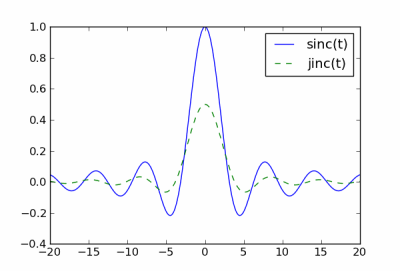# Jinc function

This afternoon I ran across the jinc function for the first time.

The sinc function is defined byThe jinc function is defined analogously bywhere J1 is a Bessel function. Bessel functions are analogous to sines, so the jinc function is analogous to the sinc function.

Here’s what the sinc and jinc functions look like.The jinc function is not as common as the sinc function. For example, both Mathematica and SciPy have built-in functions for sinc but not for jinc. [There are actually two definitions of sinc. Mathematica uses the definition above, but SciPy uses sin(πt)/πt. The SciPy convention is more common in digital signal processing.]

As I write this, Wikipedia has an entry for sinc but not for jinc. Someone want to write one?

For small t, jinc(t) is approximately cos(t/2) / 2. This approximation has error O(t4), so it’s very good for small t, useless for large t.For large values of t, jinc(t) is like a damped, shifted cosine. Specifically,with an error that decreases like O( |t|-2 ).Like the sinc function, the jinc function has a simple Fourier transform. Both transforms are zero outside the interval [-1, 1]. Inside this interval, the transform of sinc is a constant, √(π/8). On the same interval, the transform of jinc is √(2/π) √(1 – ω2).

## 6 thoughts on “Jinc function”

1. This brings to mind that the Fourier transform of a sinc function takes the value one for all frequencies below a cutoff, and zero for higher frequencies. Hence, sinc is used to construct an ideal low-pass filter (well, I think it’s mostly used in conjunction with a window function, like the Blackman window, and therefore somewhat less than ideal). It may be obvious to others, coming from different backgrounds, but this post has made me curious about the jinc function as it relates to signal processing. I wonder if someone could whip up a Bode plot.

2. Victor

The jinc function is mainly used in 2D Fourier transforms of a circular aperture, like the sinc function for a square aperture.

3. First time I see this function and I’m already in love with it.

4. Hi, thanks for this post (and the rest of the blog, and your math-related twitter accounts, that a huge amount of work!). I found it interesting. In my mathematical methods for physics course, our professor told us that sine and consine are functions defined by their properties such as being solution of a certain differential equation (harmonic oscillator, for instance). The same applies to Bessel’s functions, since they are solution to other differential equation (Bessel’s equation of course). He kind of told us that both differential equations are similiar in a certain sense, and that this fact explains, in a certain sense, the similarity between Bessel’s and circular trigonometric functions :) hope that was comprehensible, I’m not english native speaker (nor writer lol) and I only remember the intuition behind the idea he told us.

5. J D Sahr

(1) When the jinc() function is used in physics, it arises from cylindrical geometries, and it is not usual for the argument have a negative value.

(2) On the other hand, J_1(ka) can actually be evaluated for ka < 0.

6. Ben Willetts

Just what I was looking for. The “Jinc” function appeared when I was researching elliptical radiators.